## Tuesday, May 10, 2011

### Alec's Algebra Post

Solving One Step Algebra Equation
1. Isolate Variable
2.Cancel constant using the opposite integer
3.Balance it out by doing the same thing on the other side
4.Verify- solve to equalitySolving the question using Alge TilesSolving Two Step Algebra Equation
1. Isolate the variable (the unknown number in an algebra question)
2. Cancel the constant (the integer in an algebra question)
3. Balance by doing the same thing on one side and the other side of the equal sign
4. Simplify the variable
5. Repeat step #3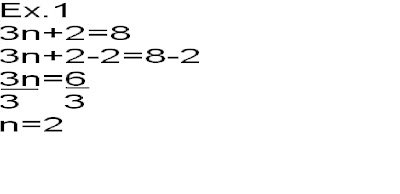Solving using Alge Tiles## Monday, May 9, 2011

### Paulo's Algebra Post

One Step Algebra Equation:

Variable: is a letter that represents an unknown number in algebra.( q, x, z, t)

Constant
: is the integer in an expression or equation.( x +6 )

Expression
: is a pattern.( 2x )

Equation
:has an equal sign. only one possible answer.( 2x=6)

-Isolate the variable
-Cancel using zero pair for the constant
-Balance on the other side of the equal signSubtractionSolving Addition/Subtraction One Step Equations Using Algebraic Tiles:
Isolate the variable
Cancel the constant using a zero pair
Balance
Verify to checkSubtraction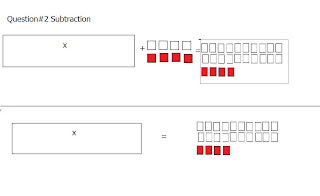Solving Multiplication/Dividing One Step Equations:
Isolate the variable
Cancel using the opposite
multiply or divide
Balance
Verify

Solving Multiplication/Dividing One Step Equations Using Algebraic Tiles:
Isolate the variable
Simplify the variable

Balance
Verify

Multiplication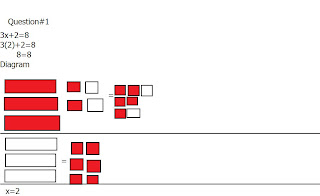Division### Roemer's AlgebraPost

Solving One Step Algebra Questions:
1. Isolate the variable. (Unkown number in the question)
2.Cancel the constant(Integer in Algebra question)

3.Balance it out by doing the same thing on both sides of the equal side.

Solving Two Step Algebra Questions:

1. Isolate the variable.
2. Cancel the constant
3. Balance it out by doing the same thing on both sides of the equal side.
5. Repate #3

### Cathlene's Algebra Post

One Step Equation #1

One Step Equation #2

One Step Equation #3

One Step Equation #4

One Step Equation #5

Two Step Equation #1

Two Step Equation #2

Two Step Equation #3

### Ryan's Algebra Post

Terms
Variable - is a letter that represents an unknown number in algebra (ex. q, x, z, t)
Constant - is the integer in an expression or equation (ex. x +6)
Expression - is a pattern; has multiple or infinite answers (ex. 2x)
Equation - has an equal sign; only one possible answer (ex. 2x = 6)

One Step Equations
Steps for Completing Equations
1. Isolate variable - get rid of constant
2. Cancel using opposite; use a zero pair for constant
3. Balance - do the same on other side of the equation
4. Verify - solve to equality

Subtraction

Subtraction Using Alge - Tiles

Multiplication

Multiplication Using Alge - Tiles

Division

Division Using Alge - Tiles

Two Step Equations
Steps for Completing Equations
1. Isolate variable - get rid of constant
2. Cancel using opposite; use a zero pair for constant
3. Balance - do the same on other side of the equation
4. Verify - solve to equality
Division

Division Using Alge - Tiles
COMING SOON.. ;)

Multiplication

Multiplication Using Alge - Tiles
COMING SOON.. ;)

### Jayvee's Algebra Post

How to Solve One Step Algebra Questions:
1) Isolate the variable (the unknown number in an algebra question)
2) Cancel the constant (the integer in an algebra question)
3) Balance by doing the same thing on one side and the other side of the equal sign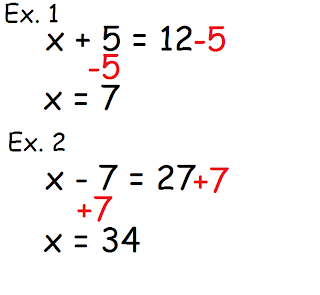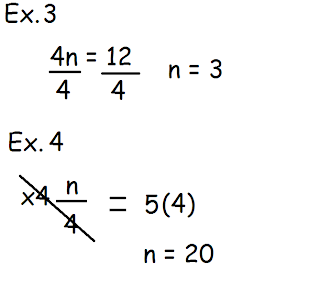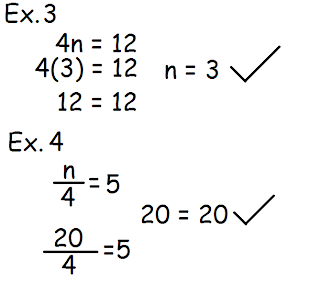Using Alge-Tiles: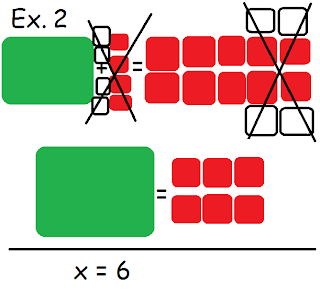How to Solve Two Step Algebra Questions:
1) Isolate the variable (the unknown number in an algebra question)
2) Cancel the constant (the integer in an algebra question)
3) Balance by doing the same thing on one side and the other side of the equal sign
4) Simplify the variable
5) Repeat step #3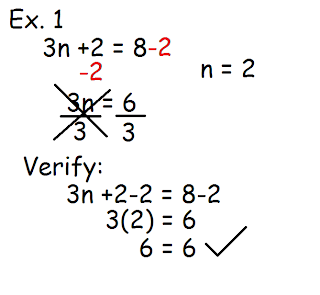Using Alge-Tiles:## Sunday, May 8, 2011

### Melanie's Algebra Post

Some terms worth remembering:
• variable: is a letter that represents an unknown number in algebra (ex: a, q, t, k)
• constant: is the integer in an expression or equation (ex: x +6)
• expression: is a pattern and has many different answers (ex: 2x)
• equation: has an equals sign and has only one possible answer (ex: 2x=6)

How to solve One Step Algebra Equations:

1) Isolate the variable
2) Cancel using the opposite (cancel the constant using a zero pair)
3) Balance both sides (do the same on both sides of the equal sign)
4) Verify to solve equality

** Here are some examples of different types of One Step Algebra Equations: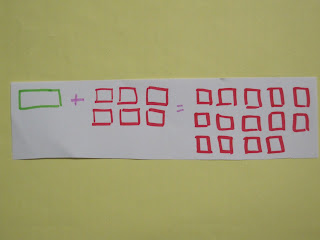Subtraction (with Alge-tiles):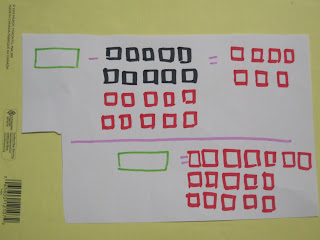Multiplication:Division: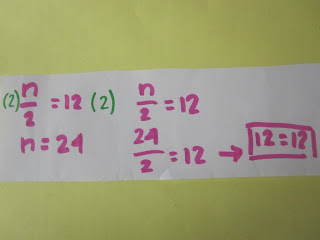How to solve Two Step Algebra Equations:

1) Isolate the variable
2) Cancel the constant
3) Balance both sides of the equals sign
4) Simplify the variable
5) Balance both sides of the equals sign again
6) Verify to check

** Here are some examples of Two Step Algebra Equations:

Multiplication:Division: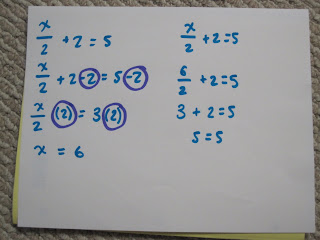### Karen's Algebra Post

One-Step Algebra Equations

Steps to solving One Step Algebra Equations:
1: Isolate the variable (variable- a letter representing an unknown/unlike number)
2: Cancel using the opposite; if there's a constant use zero pair
(constant- the integer in an algebraic expression or equation)
3: Balance, do the same on the other side of the equal sign
4: Verify to check- solve to equality

Here are some examples:
A number and 2 is 5. (n + 2 = 5)The difference of a number and 10 is -21. (n - 10 = -21)Five times a number is 20. (5 x k = 20/ 5k = 20)Alge-Tile Example:A number is divided by 8 is -4. (x ÷ 8 = -4)Two-Step Algebra Equation

Steps to solving Two Step Algebra Equations:
1: Isolate the variable
2: Cancel the constant
3: Balance
4: Simplify the variable
5: Balance again
6: Verify to check

Multiplication
Ten times a number and 3 is 43. (10g + 3 = 43)Division
A number is divided by 3 with 5 is 19.Here are somethings to help: